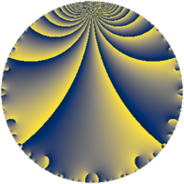# Properties

 Label 507.4.bLevel $507$ Weight $4$ Character orbit 507.b Rep. character $\chi_{507}(337,\cdot)$ Character field $\Q$ Dimension $76$ Newform subspaces $11$ Sturm bound $242$ Trace bound $17$

# Related objects

## Defining parameters

 Level: $$N$$ $$=$$ $$507 = 3 \cdot 13^{2}$$ Weight: $$k$$ $$=$$ $$4$$ Character orbit: $$[\chi]$$ $$=$$ 507.b (of order $$2$$ and degree $$1$$) Character conductor: $$\operatorname{cond}(\chi)$$ $$=$$ $$13$$ Character field: $$\Q$$ Newform subspaces: $$11$$ Sturm bound: $$242$$ Trace bound: $$17$$ Distinguishing $$T_p$$: $$2$$, $$5$$

## Dimensions

The following table gives the dimensions of various subspaces of $$M_{4}(507, [\chi])$$.

Total New Old
Modular forms 196 76 120
Cusp forms 168 76 92
Eisenstein series 28 0 28

## Trace form

 $$76 q - 296 q^{4} + 684 q^{9} + O(q^{10})$$ $$76 q - 296 q^{4} + 684 q^{9} + 32 q^{10} - 48 q^{12} + 304 q^{14} + 936 q^{16} - 316 q^{17} - 408 q^{22} + 80 q^{23} - 2100 q^{25} + 308 q^{29} + 528 q^{30} - 1040 q^{35} - 2664 q^{36} + 224 q^{38} + 280 q^{40} - 1296 q^{42} + 160 q^{43} + 1104 q^{48} - 3024 q^{49} + 192 q^{51} + 196 q^{53} - 208 q^{55} - 2208 q^{56} + 2340 q^{61} - 2788 q^{62} - 6012 q^{64} - 2184 q^{66} + 6348 q^{68} + 1272 q^{69} - 540 q^{74} + 480 q^{75} + 392 q^{77} - 5060 q^{79} + 6156 q^{81} + 3668 q^{82} + 1212 q^{87} - 2240 q^{88} + 288 q^{90} + 8620 q^{92} - 5520 q^{94} - 2000 q^{95} + O(q^{100})$$

## Decomposition of $$S_{4}^{\mathrm{new}}(507, [\chi])$$ into newform subspaces

Label Dim $A$ Field CM Traces $q$-expansion
$a_{2}$ $a_{3}$ $a_{5}$ $a_{7}$
507.4.b.a $2$ $29.914$ $$\Q(\sqrt{-3})$$ None $$0$$ $$-6$$ $$0$$ $$0$$ $$q-3q^{3}+8q^{4}+3\zeta_{6}q^{5}-6\zeta_{6}q^{7}+\cdots$$
507.4.b.b $2$ $29.914$ $$\Q(\sqrt{-1})$$ None $$0$$ $$-6$$ $$0$$ $$0$$ $$q-3q^{3}+8q^{4}-6iq^{5}-iq^{7}+9q^{9}+\cdots$$
507.4.b.c $2$ $29.914$ $$\Q(\sqrt{-1})$$ None $$0$$ $$6$$ $$0$$ $$0$$ $$q+3iq^{2}+3q^{3}-q^{4}-9iq^{5}+9iq^{6}+\cdots$$
507.4.b.d $2$ $29.914$ $$\Q(\sqrt{-1})$$ None $$0$$ $$6$$ $$0$$ $$0$$ $$q+iq^{2}+3q^{3}+7q^{4}-7iq^{5}+3iq^{6}+\cdots$$
507.4.b.e $4$ $29.914$ $$\Q(\sqrt{-3}, \sqrt{-17})$$ None $$0$$ $$-12$$ $$0$$ $$0$$ $$q+\beta _{1}q^{2}-3q^{3}-9q^{4}+(-2\beta _{1}+3\beta _{2}+\cdots)q^{5}+\cdots$$
507.4.b.f $4$ $29.914$ $$\Q(i, \sqrt{14})$$ None $$0$$ $$-12$$ $$0$$ $$0$$ $$q+\beta _{2}q^{2}-3q^{3}+(-7-\beta _{3})q^{4}+(7\beta _{1}+\cdots)q^{5}+\cdots$$
507.4.b.g $6$ $29.914$ 6.0.158155776.1 None $$0$$ $$18$$ $$0$$ $$0$$ $$q+\beta _{4}q^{2}+3q^{3}+(-3+\beta _{1})q^{4}+(\beta _{2}+\cdots)q^{5}+\cdots$$
507.4.b.h $8$ $29.914$ $$\mathbb{Q}[x]/(x^{8} + \cdots)$$ None $$0$$ $$-24$$ $$0$$ $$0$$ $$q-\beta _{2}q^{2}-3q^{3}+(-6+\beta _{1})q^{4}+(\beta _{2}+\cdots)q^{5}+\cdots$$
507.4.b.i $10$ $29.914$ $$\mathbb{Q}[x]/(x^{10} + \cdots)$$ None $$0$$ $$30$$ $$0$$ $$0$$ $$q+\beta _{1}q^{2}+3q^{3}+(-6+\beta _{4})q^{4}+(\beta _{1}+\cdots)q^{5}+\cdots$$
507.4.b.j $18$ $29.914$ $$\mathbb{Q}[x]/(x^{18} + \cdots)$$ None $$0$$ $$-54$$ $$0$$ $$0$$ $$q+\beta _{10}q^{2}-3q^{3}+(-4+\beta _{2})q^{4}+(2\beta _{10}+\cdots)q^{5}+\cdots$$
507.4.b.k $18$ $29.914$ $$\mathbb{Q}[x]/(x^{18} + \cdots)$$ None $$0$$ $$54$$ $$0$$ $$0$$ $$q-\beta _{9}q^{2}+3q^{3}+(-5+\beta _{2})q^{4}-\beta _{15}q^{5}+\cdots$$

## Decomposition of $$S_{4}^{\mathrm{old}}(507, [\chi])$$ into lower level spaces

$$S_{4}^{\mathrm{old}}(507, [\chi]) \cong$$ $$S_{4}^{\mathrm{new}}(13, [\chi])$$$$^{\oplus 4}$$$$\oplus$$$$S_{4}^{\mathrm{new}}(39, [\chi])$$$$^{\oplus 2}$$$$\oplus$$$$S_{4}^{\mathrm{new}}(169, [\chi])$$$$^{\oplus 2}$$